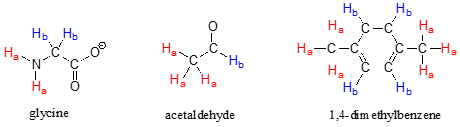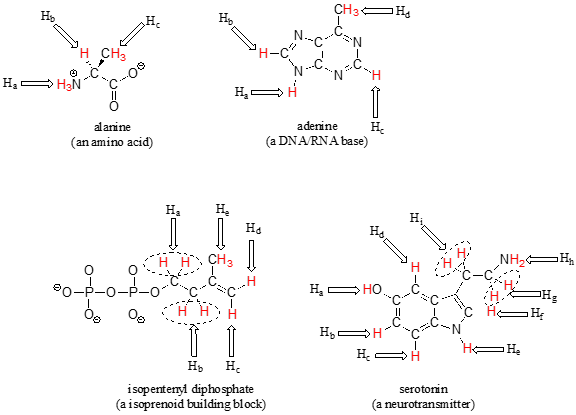# 5.3: Chemical Equivalence

$$\newcommand{\vecs}{\overset { \rightharpoonup} {\mathbf{#1}} }$$ $$\newcommand{\vecd}{\overset{-\!-\!\rightharpoonup}{\vphantom{a}\smash {#1}}}$$$$\newcommand{\id}{\mathrm{id}}$$ $$\newcommand{\Span}{\mathrm{span}}$$ $$\newcommand{\kernel}{\mathrm{null}\,}$$ $$\newcommand{\range}{\mathrm{range}\,}$$ $$\newcommand{\RealPart}{\mathrm{Re}}$$ $$\newcommand{\ImaginaryPart}{\mathrm{Im}}$$ $$\newcommand{\Argument}{\mathrm{Arg}}$$ $$\newcommand{\norm}{\| #1 \|}$$ $$\newcommand{\inner}{\langle #1, #2 \rangle}$$ $$\newcommand{\Span}{\mathrm{span}}$$ $$\newcommand{\id}{\mathrm{id}}$$ $$\newcommand{\Span}{\mathrm{span}}$$ $$\newcommand{\kernel}{\mathrm{null}\,}$$ $$\newcommand{\range}{\mathrm{range}\,}$$ $$\newcommand{\RealPart}{\mathrm{Re}}$$ $$\newcommand{\ImaginaryPart}{\mathrm{Im}}$$ $$\newcommand{\Argument}{\mathrm{Arg}}$$ $$\newcommand{\norm}{\| #1 \|}$$ $$\newcommand{\inner}{\langle #1, #2 \rangle}$$ $$\newcommand{\Span}{\mathrm{span}}$$$$\newcommand{\AA}{\unicode[.8,0]{x212B}}$$

The frequency of radiation absorbed by a proton (or any other nucleus) during a spin transition in an NMR experiment is called its 'resonance frequency'. If all protons in all organic molecules had the same resonance frequency, NMR spectroscopy but would not be terribly useful for chemists. Fortunately for us, however, resonance frequencies are not uniform for different protons in a molecule - rather, the resonance frequency varies according to the electronic environment that a given proton inhabits. In methyl acetate, for example, there are two distinct ‘sets’ of protons.The three methyl acetate protons labeled Ha above have a different resonance frequency compared to the three Hb protons, because the two sets of protons are in non-identical electronic environments: the $$H_a$$ protons are on a carbon next to a carbonyl carbon, while the $$H_b$$ protons or on a carbon next to the an oxygen. In the terminology of NMR, all three $$H_a$$ protons are chemically equivalent to each other, as are all three $$H_b$$ protons. The $$H_a$$ protons are, however, chemically nonequivalent to the $$H_b$$ protons. As a consequence, the resonance frequency of the $$H_a$$ protons is different from that of the $$H_b$$ protons. For now, do not worry about why the different electronic environment gives rise to different resonance frequencies - we will get to that soon.

The ability to recognize chemical equivalancy and nonequivalency among atoms in a molecule will be central to understanding NMR. Each of the molecules below contains only one set of chemically equivalent protons: all six protons on benzene, for example, are equivalent to each other and have the same resonance frequency in an NMR experiment. Notice that any description of the bonding and position of one proton in benzene applies to all five other protons as well.Each of the molecules in the next figure contains two sets of chemically equivalent protons, just like our previous example of methyl acetate, and again in each case the resonance frequency of the $$H_a$$ protons will be different from that of the $$H_b$$ protons.Take acetaldehyde as an example: a description of the bonding and position of the $$H_b$$ proton does not apply to the three $$H_a$$ protons: $$H_b$$ is bonded to an $$sp^2$$-hybridized carbonyl carbon while the $$H_a$$ protons are bonded to an $$sp^3$$-hybridized methyl carbon.

Note that while all four aromatic protons in 1,4-dimethylbenzene are chemically equivalent, its constitutional isomer 1,2 dimethylbenzene has two sets of aromatic protons in addition to the six methyl ($$H_a$$) protons. The 1,3-substituted isomer, on the other hand, has three sets of aromatic protons.In 1,2-dimethylbenzene, both $$H_b$$ protons are adjacent to a methyl substituent, while both $$H_c$$ protons are two carbons away. In 1,3-dimethylbenzene, $$H_b$$ is situated between two methyl groups, the two $$H_c$$ protons are one carbon away from a methyl group, and $$H_d$$ is two carbons away from a methyl group.

As you have probably already realized, chemical equivalence or non-equivalence in NMR is closely related to symmetry. Different planes of symmetry in the three isomers of dimethylbenzene lead to different patterns of equivalence.

Stereochemistry can play a part in determining equivalence or nonequivalence of nuclei in NMR. In the chloroethene (commonly known as vinyl chloride, the compound used to make polyvinyl chloride or PVC), $$H_a$$ and $$H_b$$ are in nonequivalent electronic environments, because $$H_a$$ is cis to the chlorine atom while Hb is trans. Likewise, Ha and Hb in chlorocyclopropane are nonequivalent due to their positions either on the same or opposite side of the ring relative to chlorine.If you think back to our discussion of prochirality in section 3.11, you should recognize that the $$H_a$$ and $$H_b$$ protons in the examples above are diastereotopic pairs.

Most organic molecules have several sets of protons in different chemical environments, and each set will have a different resonance frequency in $$^1H$$-NMR spectroscopy. Below we see some examples of multiple sets of protons in biological molecules.##### Exercise 5.3.1

How many sets of equivalent protons do the following molecules contain?This page titled 5.3: Chemical Equivalence is shared under a CC BY-NC-SA 4.0 license and was authored, remixed, and/or curated by Tim Soderberg via source content that was edited to the style and standards of the LibreTexts platform; a detailed edit history is available upon request.